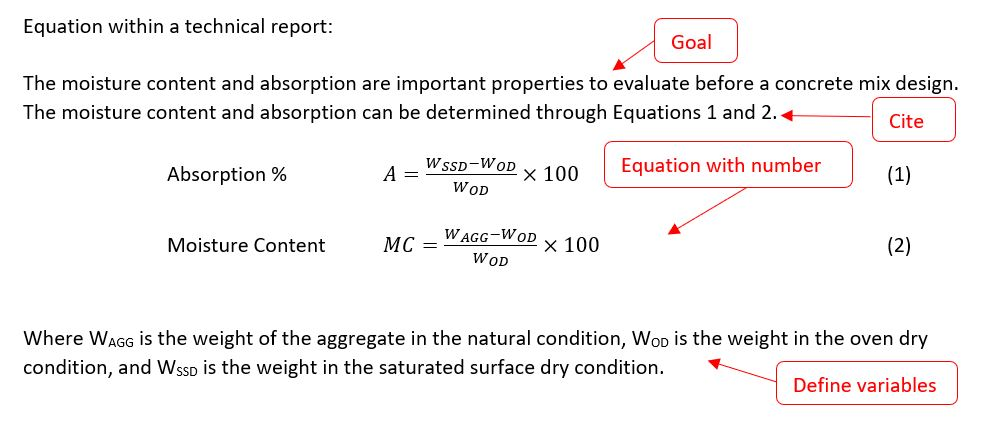## Equations - Mizzou Engineering

Often in technical communication you will need to present a mathematical equation. Learn about the proper citing and formatting of equations. Also learn about the proper use of significant figures and how to calculate the percent difference.

##### Citations

Like figures and tables, equations are numbered sequentially through the report. The number can include the chapter number. However, for equations the number is to the right of the equation and a caption is not used.

##### Equation Format

Equations themselves will often be written in an equation editing tool. To include the equation in the report, follow the following process:

1. State the goal of the equation
2. Cite the equation
3. Display the equation in symbolic form with the equation number on the right
4. Define the variables and inputs

Helpful hints for using the equation editor in Word can be found here.

List of standard equations in mechanical engineering can be found here.